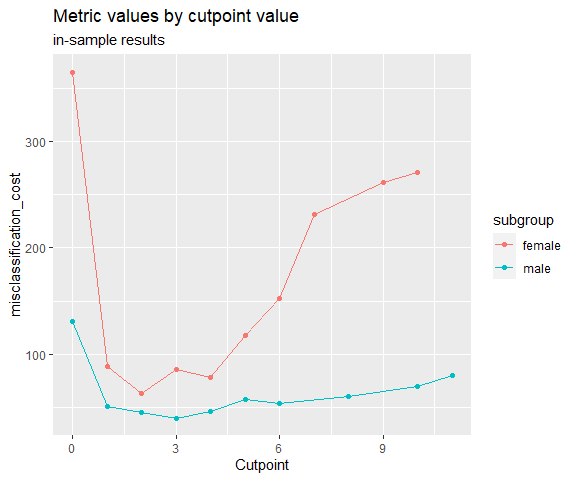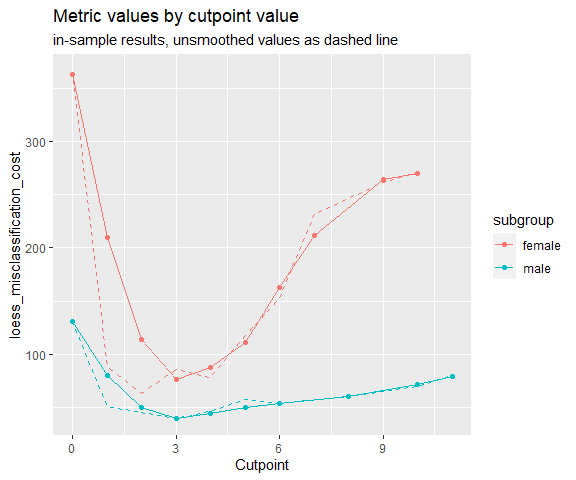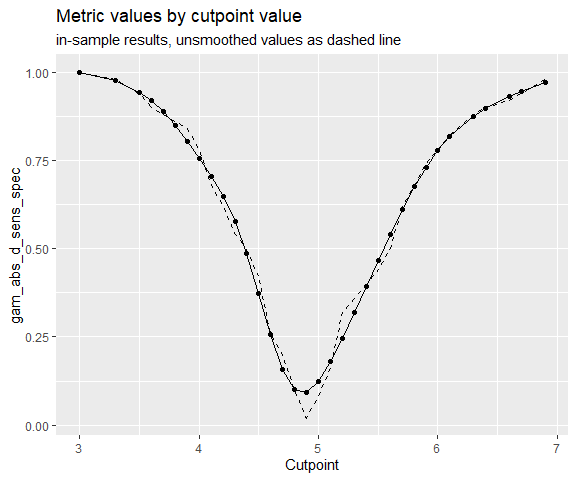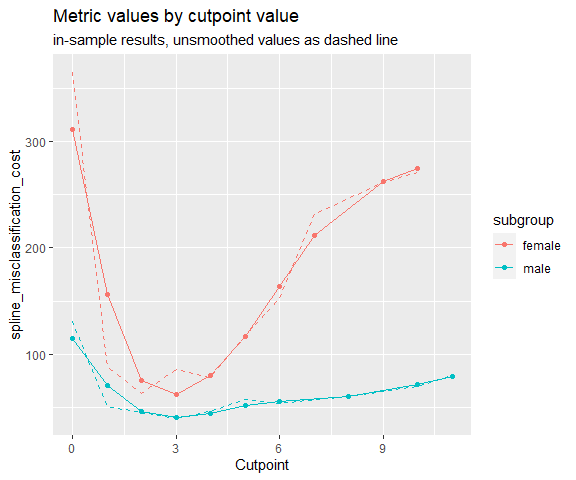# More robust cutpoint estimation methods

## Bootstrapped cutpoints

It has been shown that bagging can substantially improve performance of a wide range of types of models in regression as well as in classification tasks. This method is available for cutpoint estimation via the maximize_boot_metric and minimize_boot_metric functions. If one of these functions is used as method, boot_cut bootstrap samples are drawn, the cutpoint optimization is carried out in each one and a summary (e.g. the mean) of the resulting optimal cutpoints on the bootstrap samples is returned as the optimal cutpoint in cutpointr. Note that if bootstrap validation is run, i.e. if boot_runs is larger zero, an outer bootstrap will be executed. In the bootstrap validation routine boot_runs bootstrap samples are generated and each one is again bootstrapped boot_cut times. This may lead to long run times, so activating the built-in parallelization may be advisable.

The advantages of bootstrapping the optimal cutpoint are that the procedure doesn’t possess parameters that have to be tuned, unlike the LOESS smoothing, that it doesn’t rely on assumptions, unlike the Normal method, and that it is applicable to any metric that can be used with minimize_metric or maximize_metric, unlike the Kernel method. Furthermore, like Random Forests cannot be overfit by increasing the number of trees, the bootstrapped cutpoints cannot be overfit by running an excessive amount of boot_cut repetitions.

library(cutpointr)
set.seed(100)
cutpointr(suicide, dsi, suicide, gender,
method = maximize_boot_metric,
boot_cut = 200, summary_func = mean,
metric = accuracy, silent = TRUE)
## # A tibble: 2 x 18
##   subgroup direction optimal_cutpoint method               accuracy      acc
##   <chr>    <chr>                <dbl> <chr>                   <dbl>    <dbl>
## 1 female   >=                 5.73246 maximize_boot_metric 0.956633 0.956633
## 2 male     >=                 8.41026 maximize_boot_metric 0.95     0.95
##   sensitivity specificity      AUC pos_class neg_class prevalence outcome
##         <dbl>       <dbl>    <dbl> <fct>     <fct>          <dbl> <chr>
## 1    0.444444    0.994521 0.944647 yes       no         0.0688776 suicide
## 2    0.222222    1        0.861747 yes       no         0.0642857 suicide
##   predictor grouping data               roc_curve                boot
##   <chr>     <chr>    <list>             <list>                   <lgl>
## 1 dsi       gender   <tibble [392 x 2]> <roc_cutpointr [11 x 9]> NA
## 2 dsi       gender   <tibble [140 x 2]> <roc_cutpointr [11 x 9]> NA

## LOESS smoothing for selecting a cutpoint

When using maximize_metric and minimize_metric the optimal cutpoint is selected by searching the maximum or minimum of the metric function. For example, we may want to minimize the misclassification cost. Since false negatives (a suicide attempt was not anticipated) can be regarded as much more severe than false positives we can set the cost of a false negative cost_fn for example to ten times the cost of a false positive.

opt_cut <- cutpointr(suicide, dsi, suicide, gender, method = minimize_metric,
metric = misclassification_cost, cost_fp = 1, cost_fn = 10)
## Assuming the positive class is yes
## Assuming the positive class has higher x values
plot_metric(opt_cut)As this “optimal” cutpoint may depend on minor differences between the possible cutoffs, smoothing of the function of metric values by cutpoint value might be desirable, especially in small samples. The minimize_loess_metric and maximize_loess_metric functions can be used to smooth the function so that the optimal cutpoint is selected based on the smoothed metric values. Options to modify the smoothing, which is implemented using loess.as from the fANCOVA package, include:

• criterion: the criterion for automatic smoothing parameter selection: “aicc” denotes bias-corrected AIC criterion, “gcv” denotes generalized cross-validation.
• degree: the degree of the local polynomials to be used. It can be 0, 1 or 2.
• family: if “gaussian” fitting is by least-squares, and if “symmetric” a re-descending M estimator is used with Tukey’s biweight function.
• user.span: the user-defined parameter which controls the degree of smoothing.

Using parameters for the LOESS smoothing of criterion = "aicc", degree = 2, family = "symmetric", and user.span = 0.7 we get the following smoothed versions of the above metrics:

opt_cut <- cutpointr(suicide, dsi, suicide, gender,
method = minimize_loess_metric,
criterion = "aicc", family = "symmetric",
degree = 2, user.span = 0.7,
metric = misclassification_cost, cost_fp = 1, cost_fn = 10)
plot_metric(opt_cut)The optimal cutpoint for the female subgroup changes to 3. Note, though, that there are no reliable rules for selecting the “best” smoothing parameters. Notably, the LOESS smoothing is sensitive to the number of unique cutpoints. A large number of unique cutpoints generally leads to a more volatile curve of metric values by cutpoint value, even after smoothing. Thus, the curve tends to be undersmoothed in that scenario. The unsmoothed metric values are returned in opt_cut$roc_curve in the column m_unsmoothed. ## Smoothing via Generalized Additive Models for selecting a cutpoint In a similar fashion, the function of metric values per cutpoint can be smoothed using Generalized Additive Models with smooth terms. Internally, mgcv::gam carries out the smoothing which can be customized via the arguments formula and optimizer, see help("gam", package = "mgcv"). Most importantly, the GAM can be specified by altering the default formula, for example the smoothing function could be configured to apply cubic regression splines ("cr") as the smooth term. As the suicide data has only very few unique cutpoints, it is not very suitable for showcasing the GAM smoothing, so we will use two classes of the iris data here. In this case, the purely empirical method and the GAM smoothing lead to identical cutpoints, but in practice the GAM smoothing tends to be more robust, especially with larger data. An attractive feature of the GAM smoothing is that the default values tend to work quite well and usually require no tuning, eliminating researcher degrees of freedom. library(ggplot2) exdat <- iris exdat <- exdat[exdat$Species != "setosa", ]
opt_cut <- cutpointr(exdat, Petal.Length, Species,
method = minimize_gam_metric,
formula = m ~ s(x.sorted, bs = "cr"),
metric = abs_d_sens_spec)
## Assuming the positive class is virginica
## Assuming the positive class has higher x values
plot_metric(opt_cut)## Spline smoothing for selecting a cutpoint

Again in the same fashion the function of metric values per cutpoint can be smoothed using smoothing splines. By default, the number of knots is automatically chosen using the cutpoint_knots function. That function uses stats::.nknots.smspl, which is the default in stats::smooth.spline to pick the number of knots.

Alternatively, the number of knots can be set manually and also the other smoothing parameters of stats::smooth.spline can be set as desired. For details see ?maximize_spline_metric.

opt_cut <- cutpointr(suicide, dsi, suicide, gender,
method = minimize_spline_metric, spar = 0.4,
metric = misclassification_cost, cost_fp = 1, cost_fn = 10)
## Assuming the positive class is yes
## Assuming the positive class has higher x values
## nknots: 10
## nknots: 10
plot_metric(opt_cut)### Parametric method assuming normality

The Normal method in oc_youden_normal is a parametric method for maximizing the Youden-Index or equivalently the sum of $$Se$$ and $$Sp$$. It relies on the assumption that the predictor for both the negative and positive observations is normally distributed. In that case it can be shown that

$c^* = \frac{(\mu_P \sigma_N^2 - \mu_N \sigma_P^2) - \sigma_N \sigma_P \sqrt{(\mu_N - \mu_P)^2 + (\sigma_N^2 - \sigma_P^2) log(\sigma_N^2 / \sigma_P^2)}}{\sigma_N^2 - \sigma_P^2}$

where the negative class is normally distributed with $$\sim N(\mu_N, \sigma_N^2)$$ and the positive class independently normally distributed with $$\sim N(\mu_P, \sigma_P^2)$$ provides the optimal cutpoint $$c^*$$ that maximizes the Youden-Index. If $$\sigma_N$$ and $$\sigma_P$$ are equal, the expression can be simplified to $$c^* = \frac{\mu_N + \mu_P}{2}$$. However, the oc_youden_normal method in cutpointr always assumes unequal standard deviations. Since this method does not select a cutpoint from the observed predictor values, it is questionable which values for $$Se$$ and $$Sp$$ should be reported. Here, the Youden-Index can be calculated as

$J = \Phi(\frac{c^* - \mu_N}{\sigma_N}) - \Phi(\frac{c^* - \mu_P}{\sigma_P})$

if the assumption of normality holds. However, since there exist several methods that do not select cutpoints from the available observations and to unify the reporting of metrics for these methods, cutpointr reports all metrics, e.g. $$Se$$ and $$Sp$$, based on the empirical observations.

cutpointr(suicide, dsi, suicide, gender, method = oc_youden_normal)
## Assuming the positive class is yes
## Assuming the positive class has higher x values
## # A tibble: 2 x 18
##   subgroup direction optimal_cutpoint method           sum_sens_spec      acc
##   <chr>    <chr>                <dbl> <chr>                    <dbl>    <dbl>
## 1 female   >=                 2.47775 oc_youden_normal       1.71618 0.895408
## 2 male     >=                 3.17226 oc_youden_normal       1.54453 0.864286
##   sensitivity specificity      AUC pos_class neg_class prevalence outcome
##         <dbl>       <dbl>    <dbl> <fct>     <fct>          <dbl> <chr>
## 1    0.814815    0.901370 0.944647 yes       no         0.0688776 suicide
## 2    0.666667    0.877863 0.861747 yes       no         0.0642857 suicide
##   predictor grouping data               roc_curve                boot
##   <chr>     <chr>    <list>             <list>                   <lgl>
## 1 dsi       gender   <tibble [392 x 2]> <roc_cutpointr [11 x 9]> NA
## 2 dsi       gender   <tibble [140 x 2]> <roc_cutpointr [11 x 9]> NA

### Nonparametric kernel method

A nonparametric alternative is the Kernel method [@fluss_estimation_2005]. Here, the empirical distribution functions are smoothed using the Gaussian kernel functions $$\hat{F}_N(t) = \frac{1}{n} \sum^n_{i=1} \Phi(\frac{t - y_i}{h_y})$$ and $$\hat{G}_P(t) = \frac{1}{m} \sum^m_{i=1} \Phi(\frac{t - x_i}{h_x})$$ for the negative and positive classes respectively. Following Silverman’s plug-in “rule of thumb” the bandwidths are selected as $$h_y = 0.9 * min\{s_y, iqr_y/1.34\} * n^{-0.2}$$ and $$h_x = 0.9 * min\{s_x, iqr_x/1.34\} * m^{-0.2}$$ where $$s$$ is the sample standard deviation and $$iqr$$ is the inter quartile range. It has been demonstrated that AUC estimation is rather insensitive to the choice of the bandwidth procedure [@faraggi_estimation_2002] and thus the plug-in bandwidth estimator has also been recommended for cutpoint estimation. The oc_youden_kernel function in cutpointr uses a Gaussian kernel and the direct plug-in method for selecting the bandwidths. The kernel smoothing is done via the bkde function from the KernSmooth package [@wand_kernsmooth:_2013].

Again, there is a way to calculate the Youden-Index from the results of this method [@fluss_estimation_2005] which is

$\hat{J} = max_c \{\hat{F}_N(c) - \hat{G}_N(c) \}$

but as before we prefer to report all metrics based on applying the cutpoint that was estimated using the Kernel method to the empirical observations.

cutpointr(suicide, dsi, suicide, gender, method = oc_youden_kernel)
## Assuming the positive class is yes
## Assuming the positive class has higher x values
## # A tibble: 2 x 18
##   subgroup direction optimal_cutpoint method           sum_sens_spec      acc
##   <chr>    <chr>                <dbl> <chr>                    <dbl>    <dbl>
## 1 female   >=                 1.18128 oc_youden_kernel       1.80812 0.885204
## 2 male     >=                 1.31636 oc_youden_kernel       1.58694 0.807143
##   sensitivity specificity      AUC pos_class neg_class prevalence outcome
##         <dbl>       <dbl>    <dbl> <fct>     <fct>          <dbl> <chr>
## 1    0.925926    0.882192 0.944647 yes       no         0.0688776 suicide
## 2    0.777778    0.809160 0.861747 yes       no         0.0642857 suicide
##   predictor grouping data               roc_curve                boot
##   <chr>     <chr>    <list>             <list>                   <lgl>
## 1 dsi       gender   <tibble [392 x 2]> <roc_cutpointr [11 x 9]> NA
## 2 dsi       gender   <tibble [140 x 2]> <roc_cutpointr [11 x 9]> NA